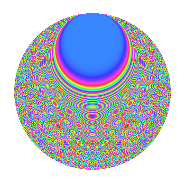# Properties

 Label 2541.2.lLevel 2541 Weight 2 Character orbit l Rep. character $$\chi_{2541}(725,\cdot)$$ Character field $$\Q(\zeta_{6})$$ Dimension 544 Sturm bound 704

# Related objects

## Defining parameters

 Level: $$N$$ = $$2541 = 3 \cdot 7 \cdot 11^{2}$$ Weight: $$k$$ = $$2$$ Character orbit: $$[\chi]$$ = 2541.l (of order $$6$$ and degree $$2$$) Character conductor: $$\operatorname{cond}(\chi)$$ = $$231$$ Character field: $$\Q(\zeta_{6})$$ Sturm bound: $$704$$

## Dimensions

The following table gives the dimensions of various subspaces of $$M_{2}(2541, [\chi])$$.

Total New Old
Modular forms 752 608 144
Cusp forms 656 544 112
Eisenstein series 96 64 32

## Trace form

 $$544q + 2q^{3} - 252q^{4} + 6q^{9} + O(q^{10})$$ $$544q + 2q^{3} - 252q^{4} + 6q^{9} - 16q^{12} + 12q^{15} - 196q^{16} + 232q^{25} - 4q^{27} - 144q^{34} - 8q^{36} + 4q^{37} - 136q^{42} - 24q^{45} + 184q^{48} + 12q^{49} - 32q^{58} + 8q^{60} + 104q^{64} - 32q^{67} + 28q^{69} + 108q^{70} + 14q^{75} - 180q^{78} + 6q^{81} + 20q^{82} - 112q^{91} - 40q^{93} + O(q^{100})$$

## Decomposition of $$S_{2}^{\mathrm{new}}(2541, [\chi])$$ into newform subspaces

The newforms in this space have not yet been added to the LMFDB.

## Decomposition of $$S_{2}^{\mathrm{old}}(2541, [\chi])$$ into lower level spaces

$$S_{2}^{\mathrm{old}}(2541, [\chi]) \cong$$ $$S_{2}^{\mathrm{new}}(231, [\chi])$$$$^{\oplus 2}$$

## Hecke Characteristic Polynomials

There are no characteristic polynomials of Hecke operators in the database